Ex.3.7 (Optional)

Chapter 3 Class 10 Pair of Linear Equations in Two Variables
Serial order wise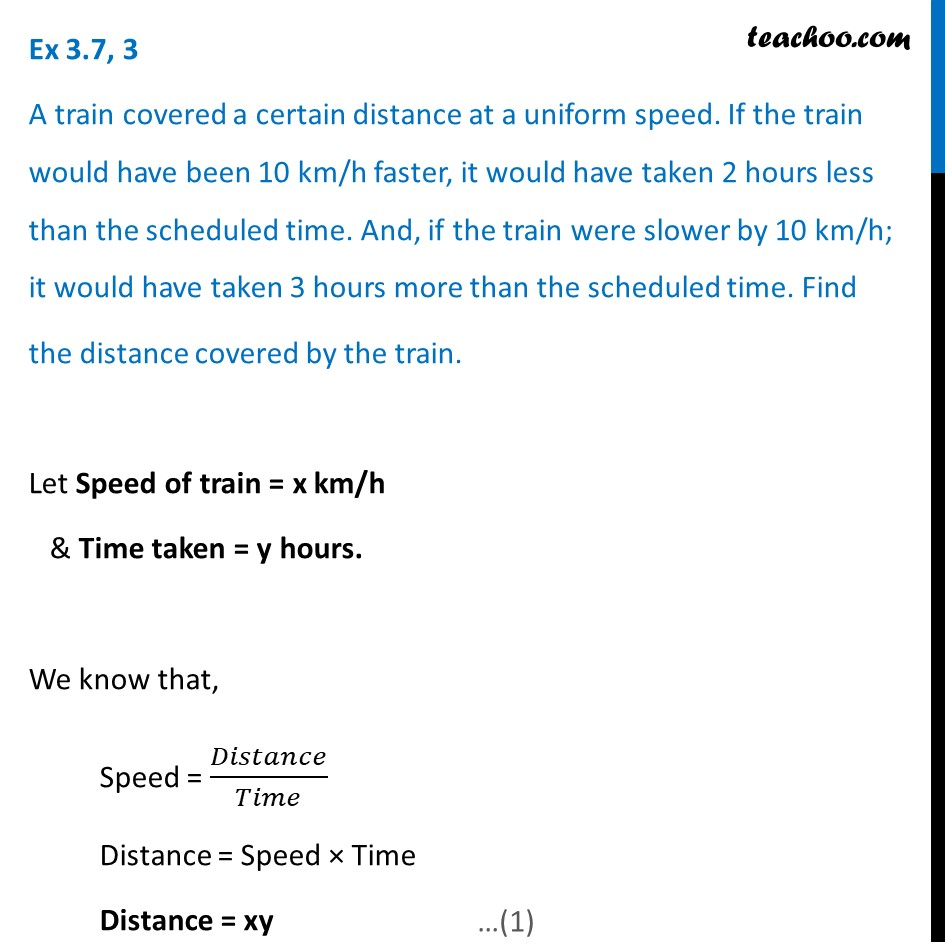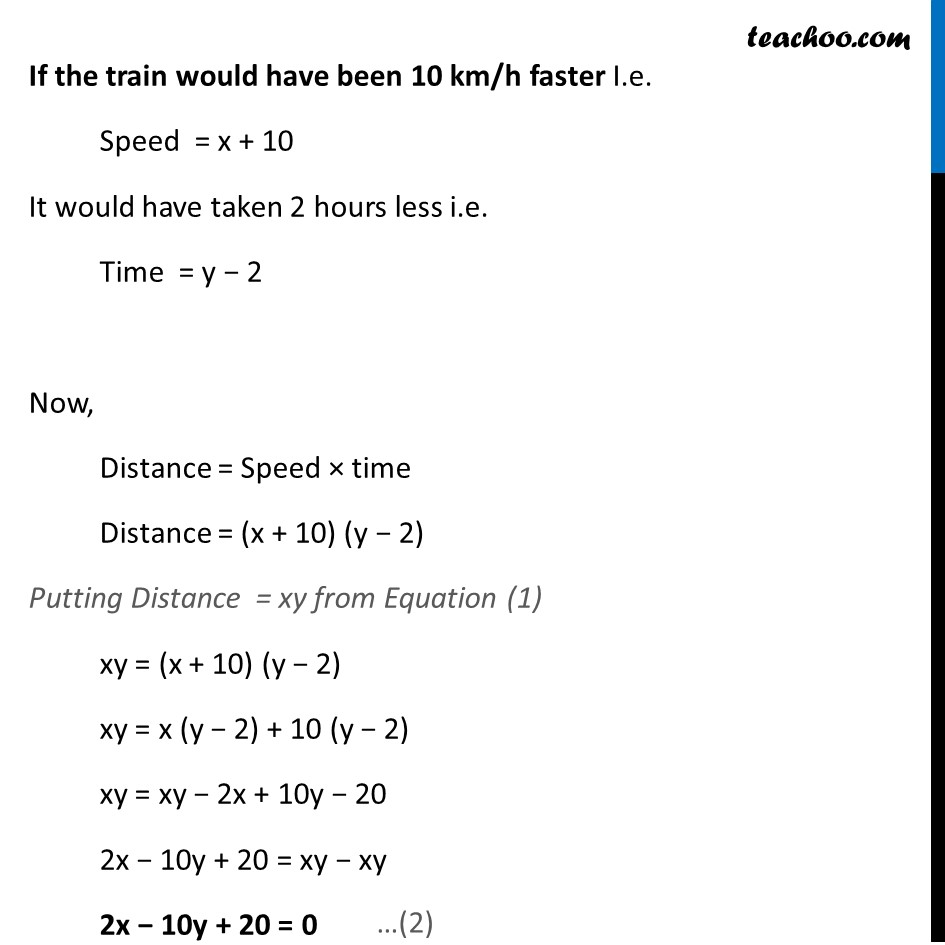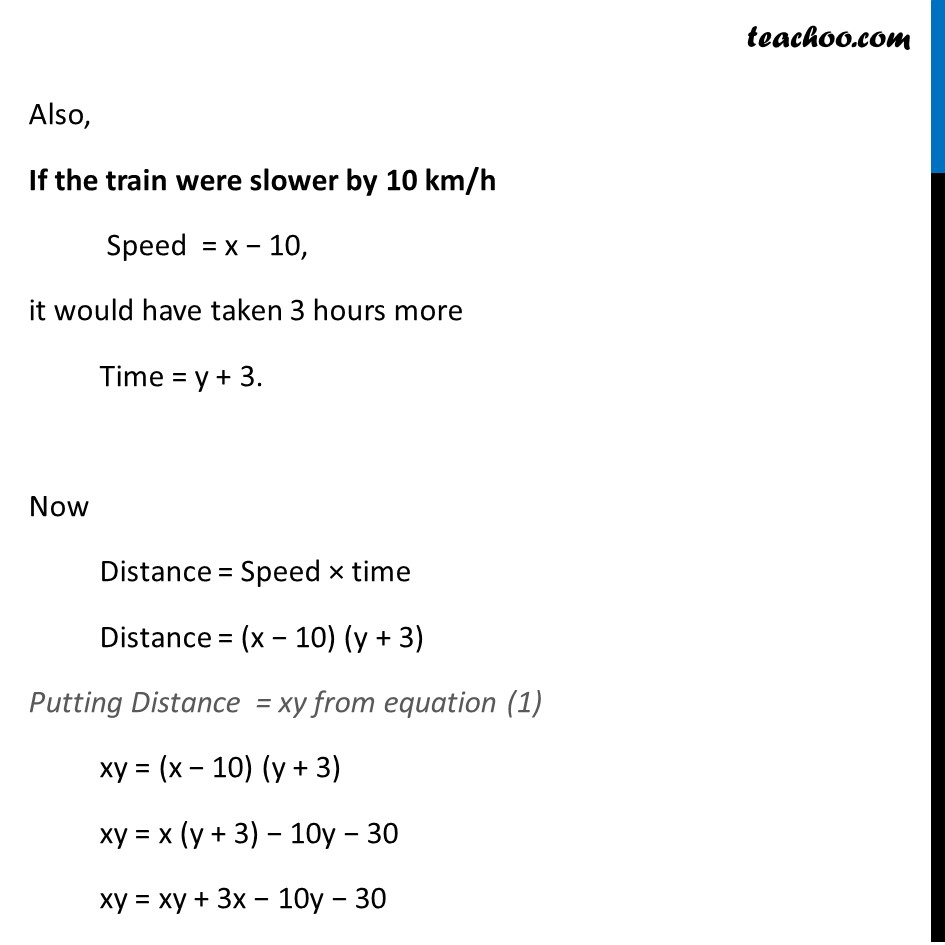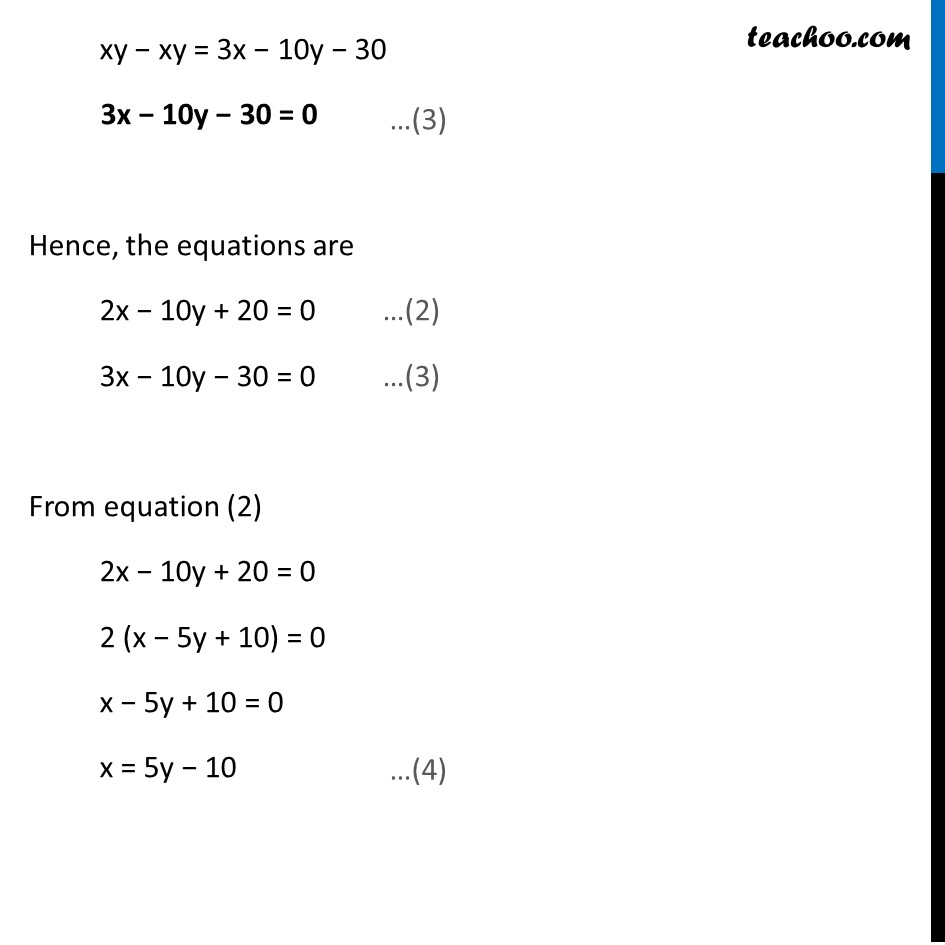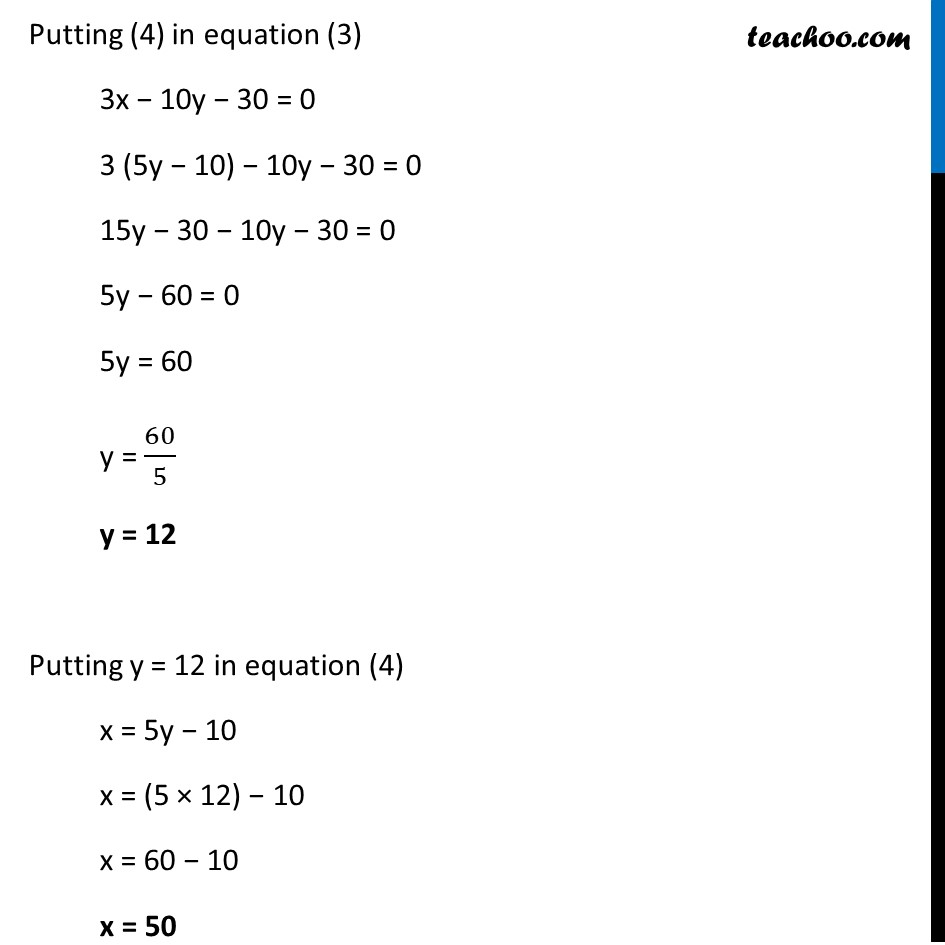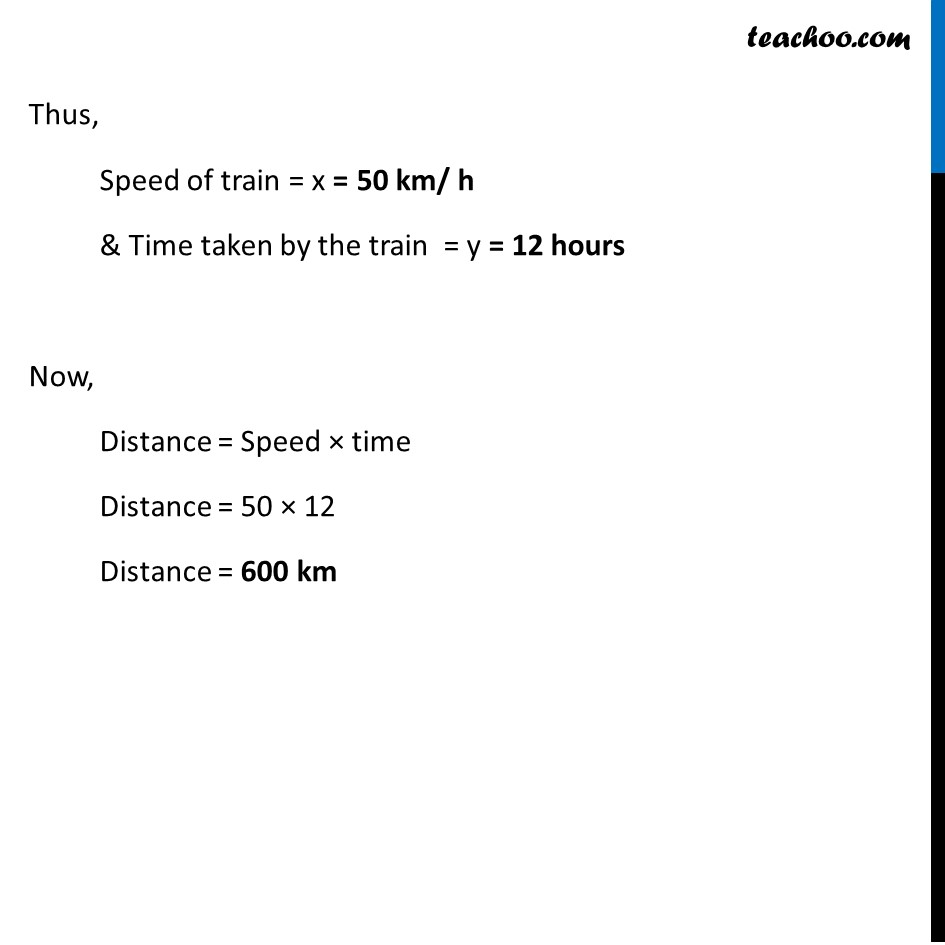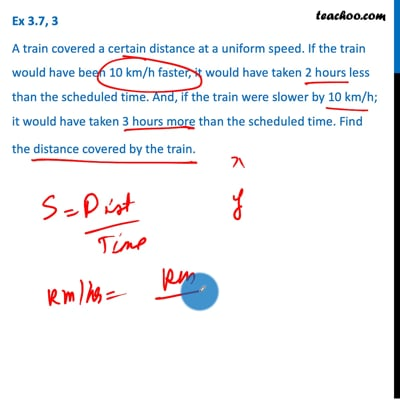This video is only available for Teachoo black users

Maths Crash Course - Live lectures + all videos + Real time Doubt solving!

### Transcript

Ex 3.7, 3 A train covered a certain distance at a uniform speed. If the train would have been 10 km/h faster, it would have taken 2 hours less than the scheduled time. And, if the train were slower by 10 km/h; it would have taken 3 hours more than the scheduled time. Find the distance covered by the train. Let Speed of train = x km/h & Time taken = y hours. We know that, Speed = 𝐷𝑖𝑠𝑡𝑎𝑛𝑐𝑒/𝑇𝑖𝑚𝑒 Distance = Speed × Time Distance = xy If the train would have been 10 km/h faster I.e. Speed = x + 10 It would have taken 2 hours less i.e. Time = y − 2 Now, Distance = Speed × time Distance = (x + 10) (y − 2) Putting Distance = xy from Equation (1) xy = (x + 10) (y − 2) xy = x (y − 2) + 10 (y − 2) xy = xy − 2x + 10y − 20 2x − 10y + 20 = xy − xy 2x − 10y + 20 = 0 Also, If the train were slower by 10 km/h Speed = x − 10, it would have taken 3 hours more Time = y + 3. Now Distance = Speed × time Distance = (x − 10) (y + 3) Putting Distance = xy from equation (1) xy = (x − 10) (y + 3) xy = x (y + 3) − 10y − 30 xy = xy + 3x − 10y − 30 xy − xy = 3x − 10y − 30 3x − 10y − 30 = 0 Hence, the equations are 2x − 10y + 20 = 0 …(2) 3x − 10y − 30 = 0 …(3) From equation (2) 2x − 10y + 20 = 0 2 (x − 5y + 10) = 0 x − 5y + 10 = 0 x = 5y − 10 Putting (4) in equation (3) 3x − 10y − 30 = 0 3 (5y − 10) − 10y − 30 = 0 15y − 30 − 10y − 30 = 0 5y − 60 = 0 5y = 60 y = 60/5 y = 12 Putting y = 12 in equation (4) x = 5y − 10 x = (5 × 12) − 10 x = 60 − 10 x = 50 Thus, Speed of train = x = 50 km/ h & Time taken by the train = y = 12 hours Now, Distance = Speed × time Distance = 50 × 12 Distance = 600 km